Home Practice
For learners and parents For teachers and schools
Textbooks
Full catalogue
Pricing SupportLog in

We think you are located in United States. Is this correct?

# 6.2 Lewis structures

## 6.2 Lewis structures (ESABS)

Lewis notation uses dots and crosses to represent the valence electrons on different atoms. The chemical symbol of the element is used to represent the nucleus and the inner electrons of the atom. To determine which are the valence electrons we look at the last energy level in the atom's electronic structure (Chapter 4). For example, chlorine's electronic structure can be written as: $$1\text{s}^{2}2\text{s}^{2}2\text{p}^{6}3\text{s}^{2}3\text{p}^{5}$$ or $$[\text{Ne}]3\text{s}^{2}3\text{p}^{5}$$. The last energy level is the third one and it contains 7 electrons. These are the valence electrons.

If we write the condensed electron configuration, then we can easily see the valence electrons.

For example:

A hydrogen atom (one valence electron) would be represented like this: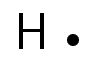A chlorine atom (seven valence electrons) would look like this:A molecule of hydrogen chloride would be shown like this: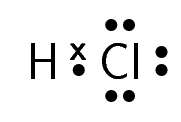The dot and cross in between the two atoms, represent the pair of electrons that are shared in the covalent bond.

Table 6.1 gives some further examples of Lewis diagrams.

 Iodine $$\text{I}_{2}$$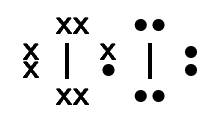Water $$\text{H}_{2}\text{O}$$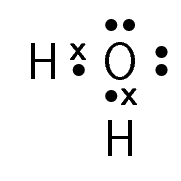Carbon dioxide $$\text{CO}_{2}$$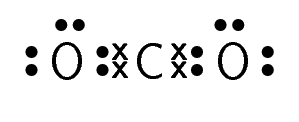Hydrogen cyanide $$\text{HCN}$$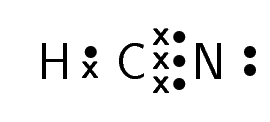Table 6.1: Lewis diagrams for some simple molecules

For carbon dioxide, you can see how we represent a double bond in Lewis notation. As there are two bonds between each oxygen atom and the carbon atom, two pairs of valence electrons link them. Similarly, hydrogen cyanide shows how to represent a triple bond.

## Lewis structures

Textbook Exercise 6.1

Represent each of the following atoms using Lewis notation:

1. beryllium
2. calcium
3. lithium
Solution not yet available.

Represent each of the following molecules using Lewis notation:

1. bromine gas $$(\text{Br}_{2})$$
2. carbon dioxide $$(\text{CO}_{2})$$

Which of these two molecules contains a double bond?

Solution not yet available.

Two chemical reactions are described below.

• Nitrogen and hydrogen react to form $$\text{NH}_{3}$$
• Carbon and hydrogen bond to form a molecule of $$\text{CH}_{4}$$

For each reaction, give:

1. the number of electrons in the outermost energy level
2. the Lewis structure of the product that is formed
3. the name of the product
Solution not yet available.

A chemical compound has the following Lewis notation:

1. How many valence electrons does element Y have?

2. What is the valency of element Y? (remember that the valency of an atom is the number of chemical bonds it can form.)

3. What is the valency of element X?

4. How many covalent bonds are in the molecule?

5. Suggest a name for the elements X and Y.

Solution not yet available.